# 数据挖掘入门系列教程（八）之使用神经网络（基于pybrain）识别数字手写集MNIST

MNIST（Mixed National Institute of Standards and Technology database）相信大家基本上都了解过他，大部分的机器学习入门项目就是它。它是一个非常庞大的手写数字数据集（官网）。里面包含了0~9的手写的数字。部分数据如下：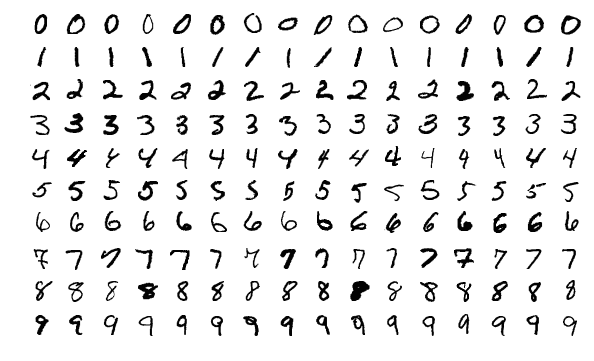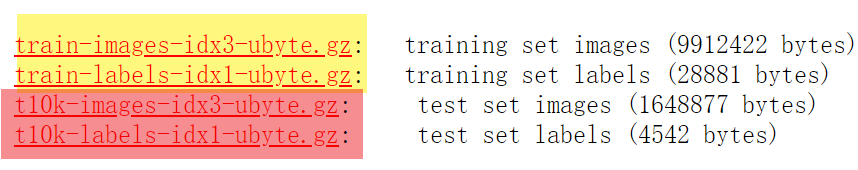## 下载数据集

import numpy as np
from sklearn.datasets import fetch_openml
# X为image数据，y为标签
X, y = fetch_openml('mnist_784', version=1, return_X_y=True)


X[X > 0 ] = 1
np.save("./Data/dataset",X)
np.save("./Data/class",y)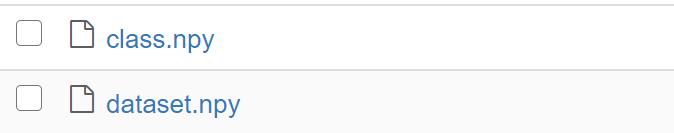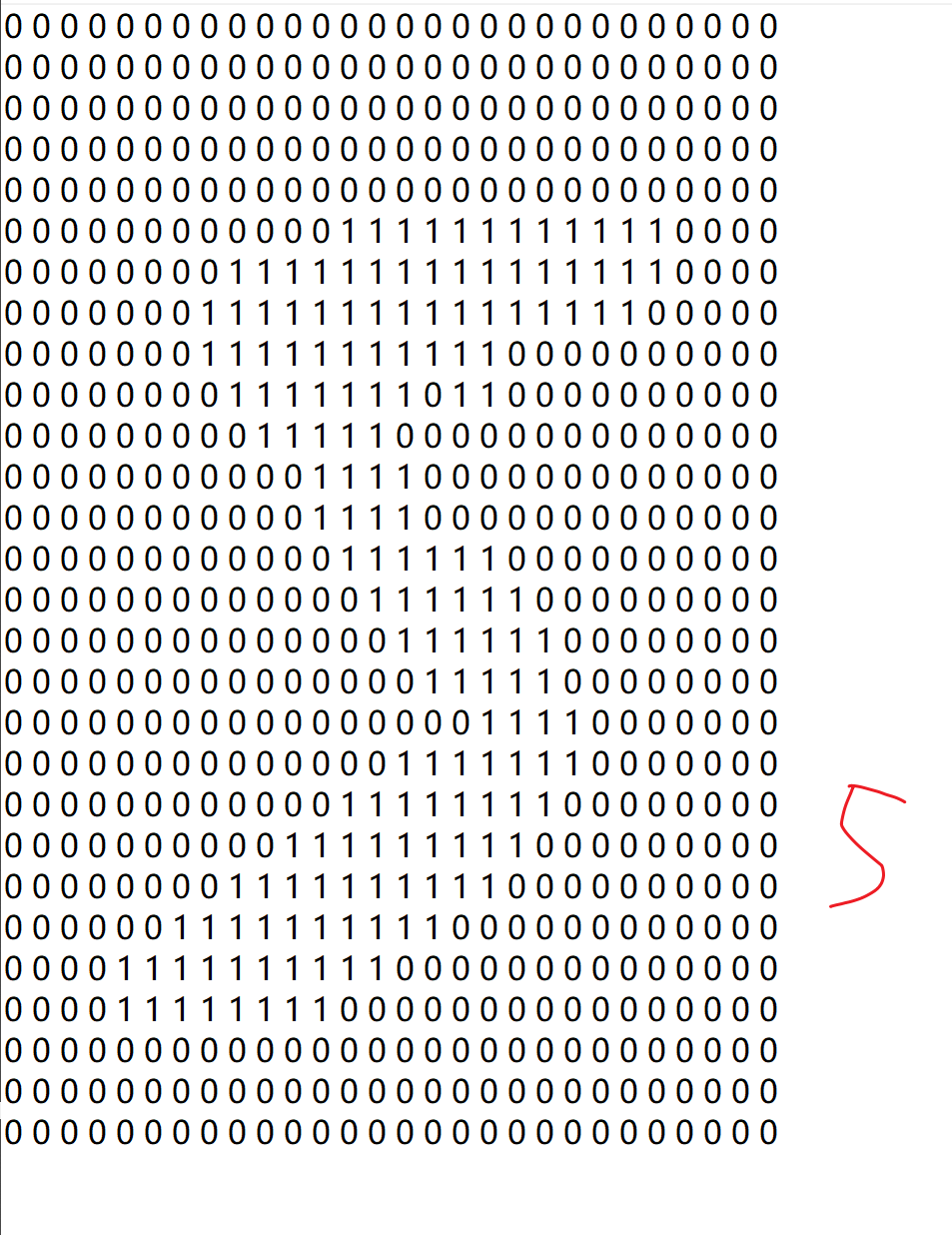## 加载数据集

import numpy as np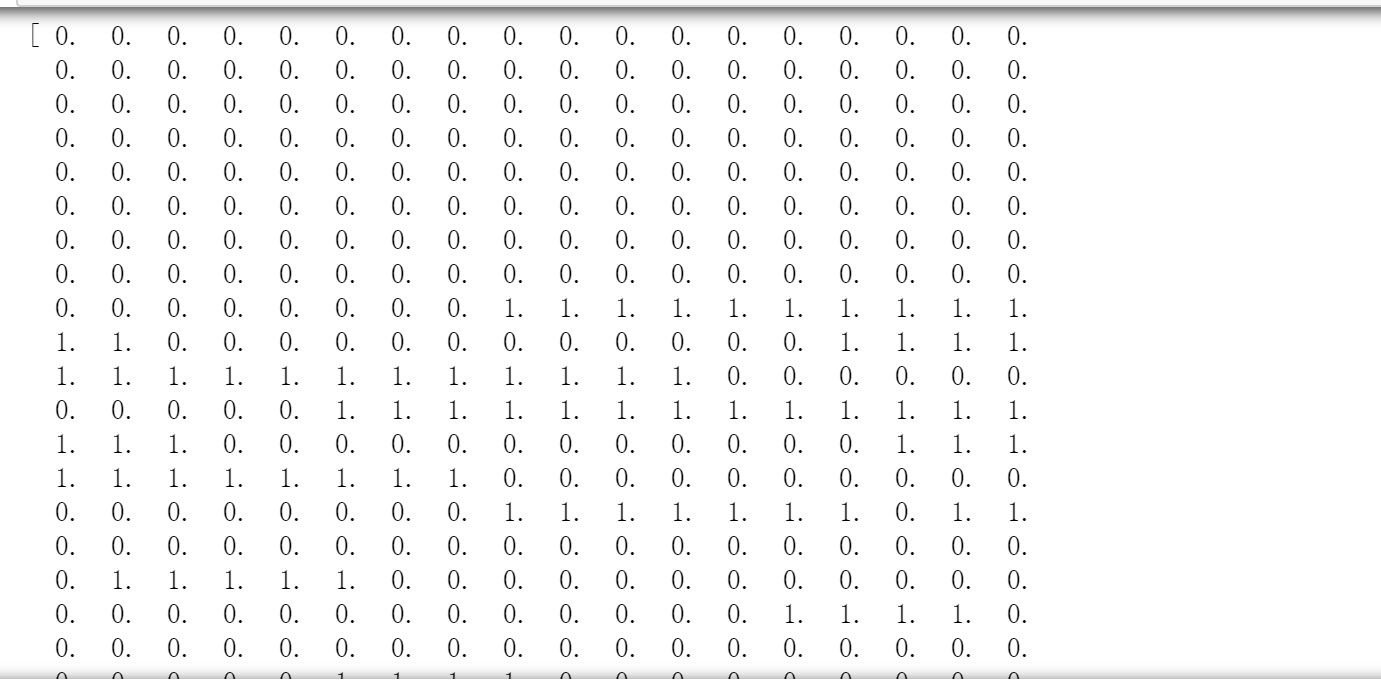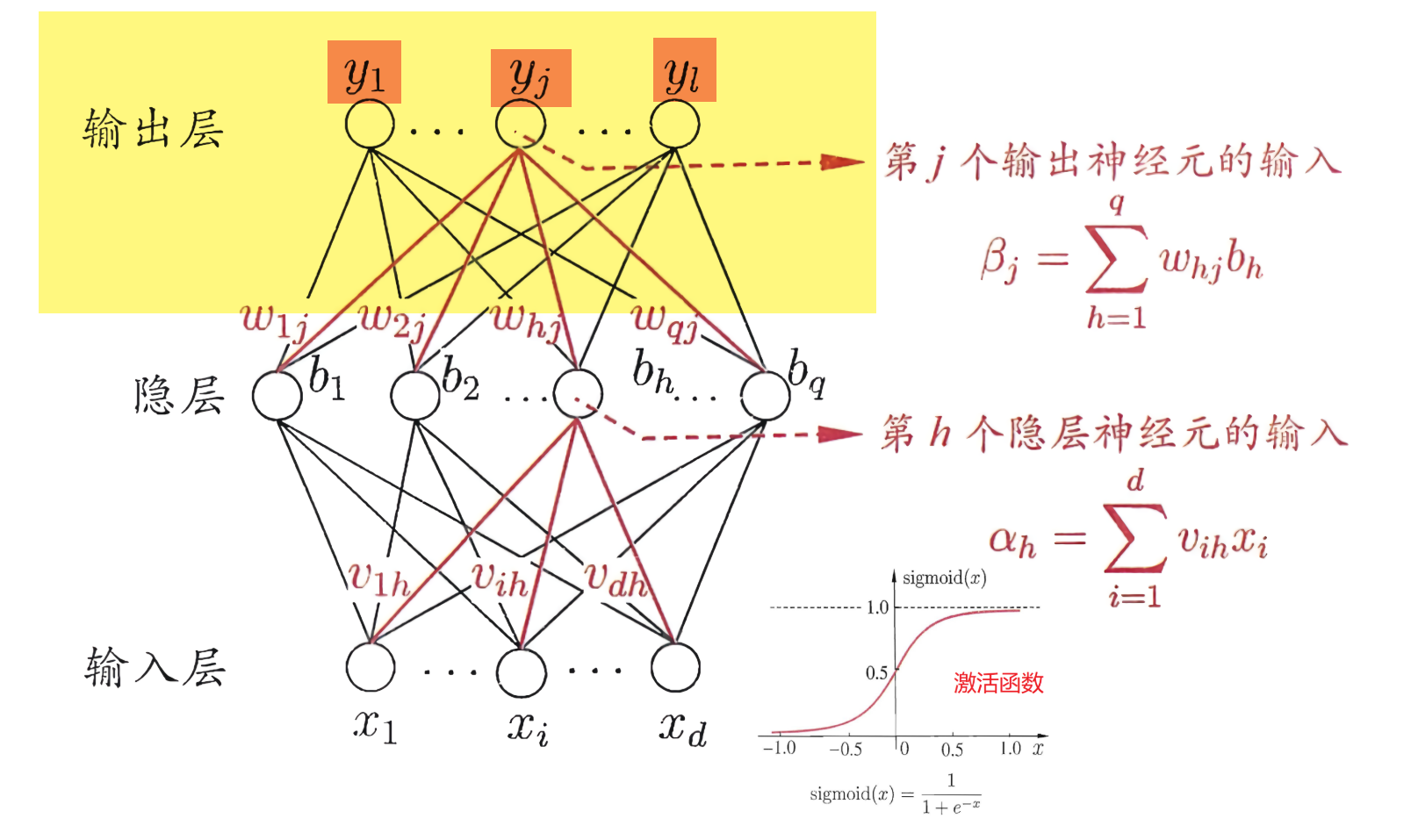One-Hot编码，又称为一位有效编码，主要是采用$N$位状态寄存器来对$N$个状态进行编码，每个状态都由他独立的寄存器位，并且在任意时候只有一位有效。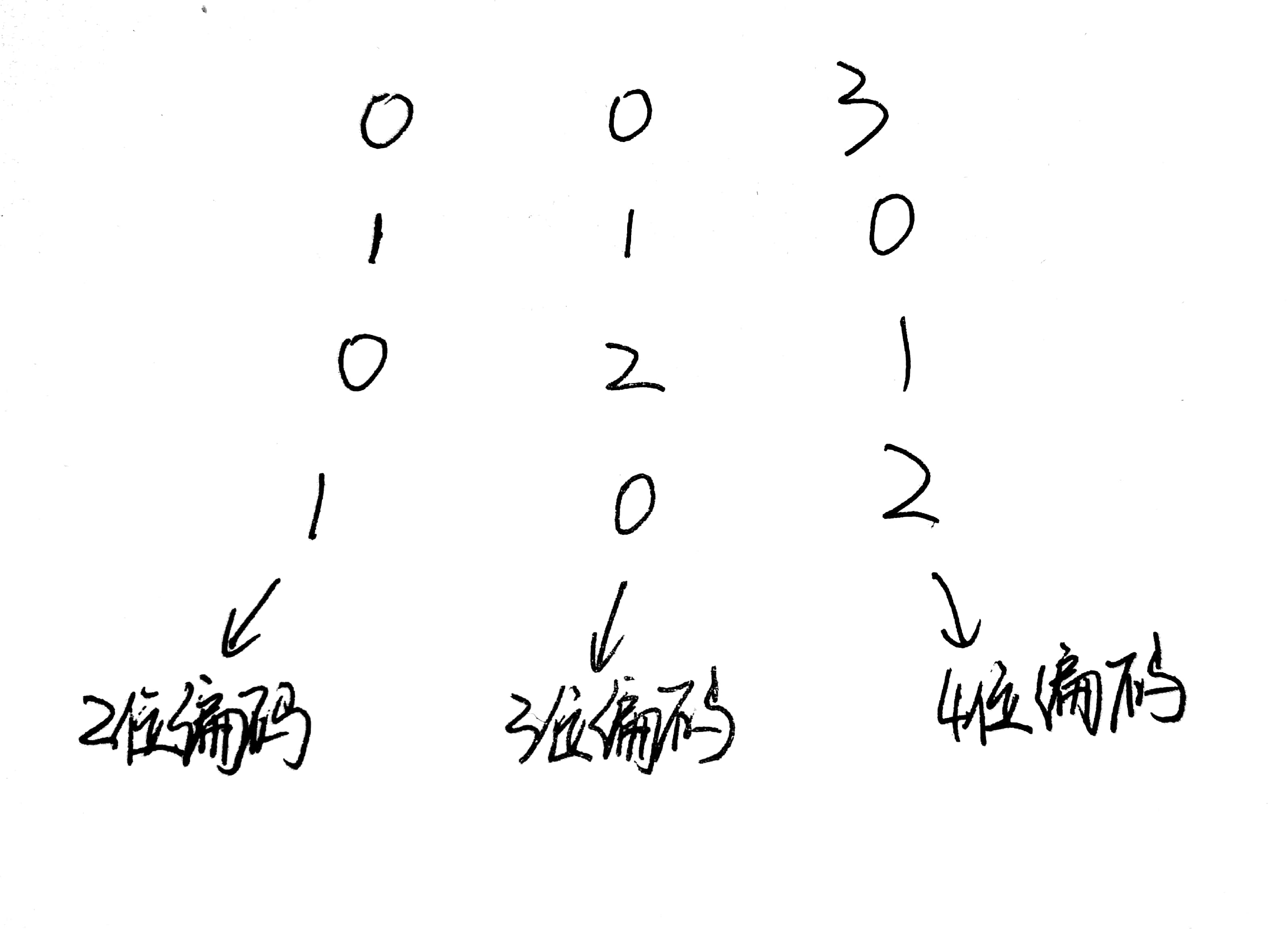from sklearn.preprocessing import OneHotEncoder
# False代表不生成稀疏矩阵
onehot = OneHotEncoder(sparse = False)
# 首先将y转成行长为7000，列长为1的矩阵，然后再进行转化。
y = onehot.fit_transform(y.reshape(y.shape,1))


from sklearn.model_selection import train_test_split
x_train,x_test,y_train,y_test = train_test_split(X,y,random_state=14)


from pybrain.datasets import SupervisedDataSet

train_data = SupervisedDataSet(x_train.shape,y.shape)
test_data = SupervisedDataSet(x_test.shape,y.shape)

for i in range(x_train.shape):
for i in range(x_test.shape):


## 构建神经网络

from pybrain.tools.shortcuts import buildNetwork
# X.shape代表属性的个数，100代表隐层中神经元的个数，y.shape代表输出
net = buildNetwork(X.shape,100, y.shape, bias=True)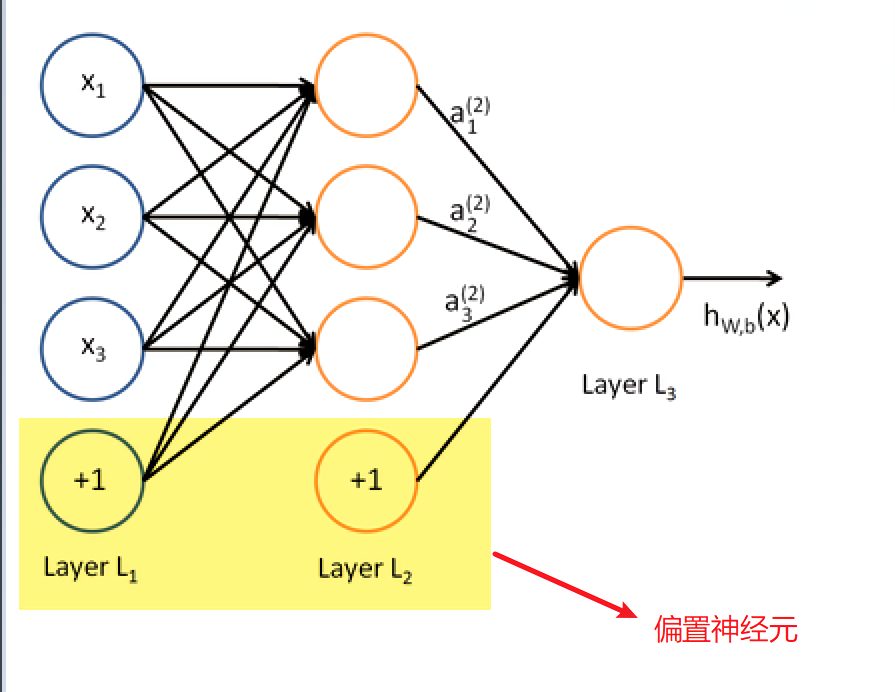## 反向传播(BP)算法

from pybrain.supervised.trainers import BackpropTrainer
trainer = BackpropTrainer(net, train_data, learningrate=0.01,weightdecay=0.01)


• net：神经网络

• train_data：训练的数据集

• learningrate：学习率，也就是下面的$\eta$，同样它可以使用lrdecay这个参数去控制衰减率，具体的就去看官网文档吧。

$\begin{equation}\begin{array}{l} \Delta w_{h j}=\eta g_{j} b_{h} \\ \Delta \theta_{j}=-\eta g_{j} \\ \Delta v_{i h}=\eta e_{h} x_{i} \\ \Delta \gamma_{h}=-\eta e_{h} \\ \end{array}\end{equation}$

• weightdecay：权重衰减，权重衰减也就是下面的$\lambda$

$\begin{equation} E=\lambda \frac{1}{m} \sum_{k=1}^{m} E_{k}+(1-\lambda) \sum_{i} w_{i}^{2} \\ \lambda \in(0,1) \end{equation}$

trainer.trainEpochs(epochs=100)


epochs也就是训练集被训练遍历的次数。

### 进行预测

predictions = trainer.testOnClassData(dataset=test_data)


predictions的部分数据，代表着测试集预测的结果：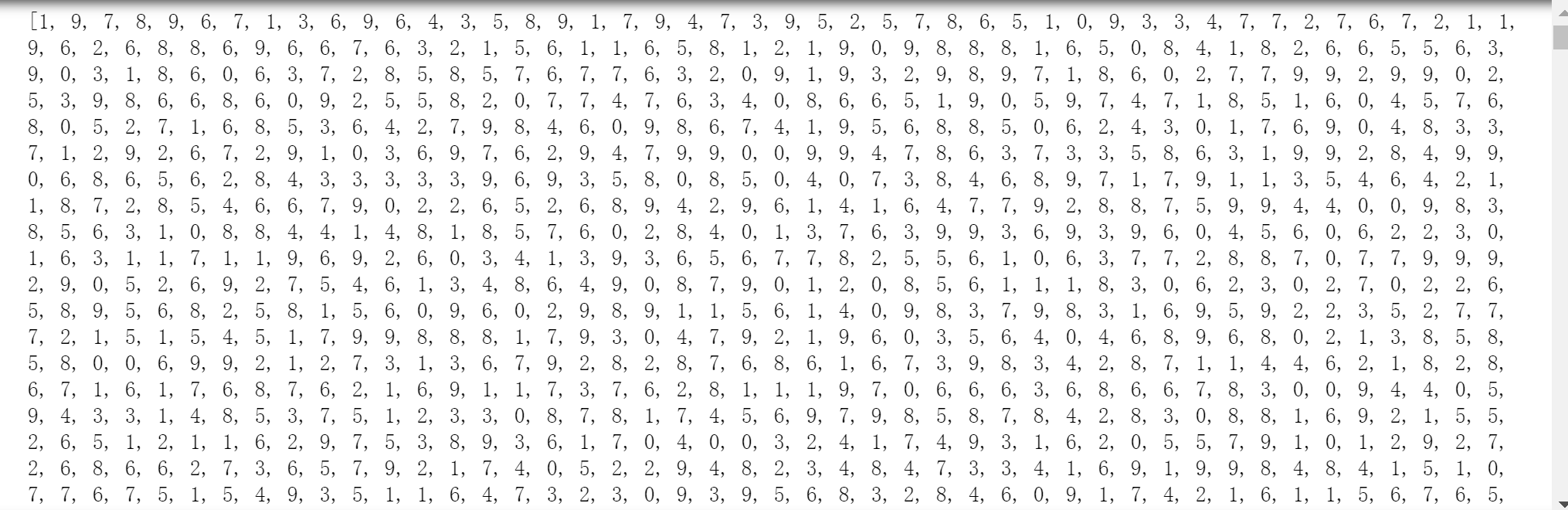## F1验证

# 取每一行最大值的索引。
y_test_arry = y_test.argmax(axis =1)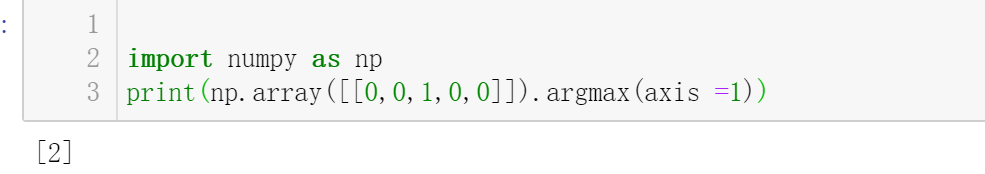from sklearn.metrics import f1_score
print("F-score: {0:.2f}".format(f1_score(predictions,y_test_arry,average='micro')))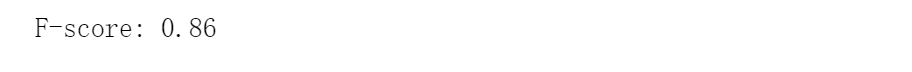## 总结

### 参考

posted @ 2020-04-05 01:36  段小辉  阅读(...)  评论(...编辑  收藏